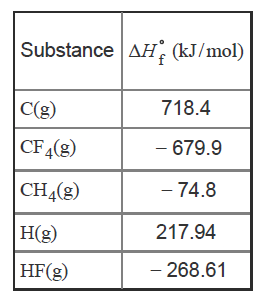# Problem: Suppose that 0.290 mol of methane, CH4(g), is reacted with 0.440 mol of fluorine, F2(g), forming CF4(g) and HF(g) as sole products. Assuming that the reaction occurs at constant pressure, how much heat is released?Express your answer to three significant figures and include the appropriate units.Use the data below to answer the questions.Keep in mind that the enthalpy of formation of an element in its standard state is zero.Imagine a hypothetical process in which the methane molecule, CH4, is "expanded," by simultaneously extending all four C−H bonds to infinity. We then have the processCH4(g) → C(g) + 4 H(g)

###### FREE Expert Solution
94% (191 ratings)View Complete Written Solution
###### Problem Details

Suppose that 0.290 mol of methane, CH4(g), is reacted with 0.440 mol of fluorine, F2(g), forming CF4(g) and HF(g) as sole products. Assuming that the reaction occurs at constant pressure, how much heat is released?

Express your answer to three significant figures and include the appropriate units.

Use the data below to answer the questions.Keep in mind that the enthalpy of formation of an element in its standard state is zero.

Imagine a hypothetical process in which the methane molecule, CH4, is "expanded," by simultaneously extending all four C−H bonds to infinity. We then have the process

CH4(g) → C(g) + 4 H(g)